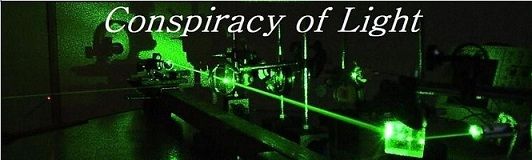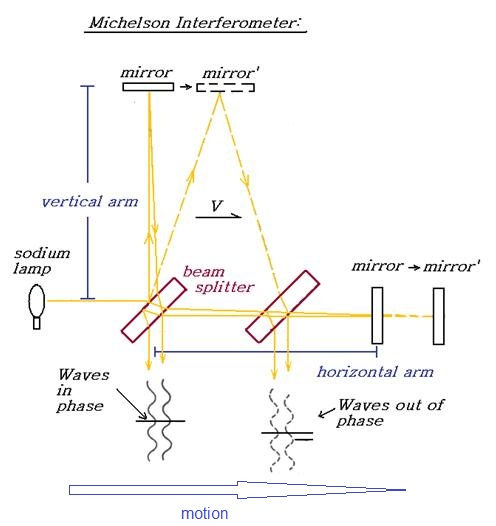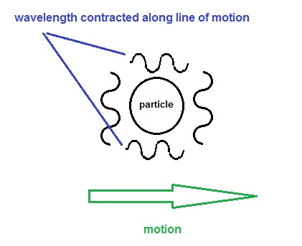The search for  new physics.

Possible Explanation for the Physical Lorentz Contraction

Doug Marett (2015)

This explanation is for the physical Lorentz contraction of Hendrik Lorentz and George Fitzgerald, as distinct from that of Einstein. Einstein did not of course believe that the Lorentz contraction was physical, but rather believed that it was an effect dependent on the perspective of the observer. However, in this discussion we will consider how the Lorentz contraction could actually be a physical effect as was believed by Lorentz, Fitzgerald, and the Maxwellians. The effect was originally criticized as being artificial or ad hoc by Popper, serving to restore agreement between theory and experiment. However, certain findings about the nature of matter in the 1920’s led to an understanding that would be entirely compatible with this original premise of a physical contraction of matter with velocity. Consider the propagation time of light in a Michelson interferometer using Lorentz’s equation incorporating the Fresnel drag coefficient:The2 way time required for a beam of light to travel perpendicular to its direction of travel at velocity V with respect to a preferred frame is:

2T = 2 x [D/(C/n – W/n^2)]

Or

If n=1, then 2T = 2D/C x 1/(SQRT(1-v^2/c^2))    see: ref

The 2-way time required for a beam of light to travel parallel under the same conditions is:

2T= D/(C/n+ v/n^2)+ D/(C/n- v/n^2)

Or

If n =1, then 2T = 2D/C x (1 + v^2/C^2)   see: ref

Where: D = the one way path length       V = velocity through space         n = refractive index of arms

C = speed of light in space     W = transverse velocity component   C – SQRT(C^2 – v^2)

The parallel beam transit time is found to be slower than the perpendicular by the factor

1/SQRT(1-V^2/C^2)           (The Lorentz factor)

The hypothesis put forward by Fitzgerald and Lorentz was that the apparatus must contract in the parallel direction by this factor to nullify the result.  But why would matter contract in the direction of motion when it experiences a velocity?

All we have to do is consider that atoms and other particles behave just like waves, and have dimensions based on their wavelength (including their bond lengths). Evidence has long suggested that atoms and particles are composed of waves, have a wavelength and a frequency, akin to photons. As is well known, electrons fired through a Young double slit will interfere with one another, just like waves of light. When Schrodinger developed his model of the atom and atomic orbitals, he was building on De Broglie’s hypothesis that particles had a wavelike essence. Schrodinger’s model, which correctly predicted the shape of atomic orbitals, was based on modelling how electromagnetic waves could be constrained to form a stable 3D structure in space. This is why when Schrodinger first invented Quantum Mechanics, he called it “Wave Mechanics.” Schrodinger was an expert in hydrodynamics, the motion of fluids, which is what made him ideally situated for figuring out the orbital problem. If the reader can accept the notion that the dimensions of matter and particles depend upon their wavelength in the vein intended by the two Nobel Laureates above, and that this wavelength depends upon the speed of light, then it is simply a matter of investigating how these wavelengths would be expected to change with motion in order to understand what the physical Lorentz contraction really is.

We know that the equation C= fl governs the properties of light - we apply this also to particles. If we consider a beam of light crossing to and fro the breadth and width of a particle moving at some velocity v, the time to pass perpendicular and parallel to its motion will be identical to that for the Michelson interferometer discussed above. Since the apparent speed of light C’ is slower along the parallel path in as measured in the moving frame, if C goes down, then following C= fl the wavelength l must contract to compensate. (Frequency cannot change as this is thermodynamically impossible) And since C slows down in the moving frame on average by the factor:

C’ = C x SQRT (1-V^2/C^2)

then the wavelength of the moving particles or atoms must also contract by the same factor

l’ = l x SQRT (1-V^2/C^2)

as measured in the moving frame of the particle, in the direction of motion.

Since an atom’s size and bond length should be defined by its wavelength, if its wavelength is shorter in the direction of motion than perpendicular to it, then the moving matter will contract exactly as predicted by FitzGerald and Lorentz. This is only true of course if an atom is composed of waves and moves with respect to a preferred frame of reference for these waves.  Proof of the existence of a physical Lorentz contraction would then give credence to these two former conditions.Any attempt to measure anisotropy in the speed of light in this manner will be impossible, since both the matter and the laser light will experience a wavelength contraction that will cancel out around the interferometer. This provides an alternative explanation for why no Michelson Morley type interferometer experiment has ever been able to detect our motion through space, because this effect will lead the moving observer to erroneously measure the speed of light to be equal in all directions, regardless of this motion.

Note: Schrodinger’s wave model of the atom was overruled by the Copenhagen Interpretation of Quantum Mechanics, largely due to the pressure of Niels Bohr. Perhaps a wave-mechanical atom was too much for the particle-ists. Schrodinger was vehemently against this re-interpretation of his work, saying:

Let me say at the outset, that in this discourse, I am opposing not a few special statements of quantum physics held today (1950s), I am opposing as it were the whole of it, I am opposing its basic views that have been shaped 25 years ago, when Max Born put forward his probability interpretation, which was accepted by almost everybody. (Schrödinger E, The Interpretation of Quantum Physics. Ox Bow Press, Woodbridge, CN, 1995).

De Broglie – Nobel Prize in Physics – 1929

Erwin Schrodinger – Nobel Prize in Physics - 1933